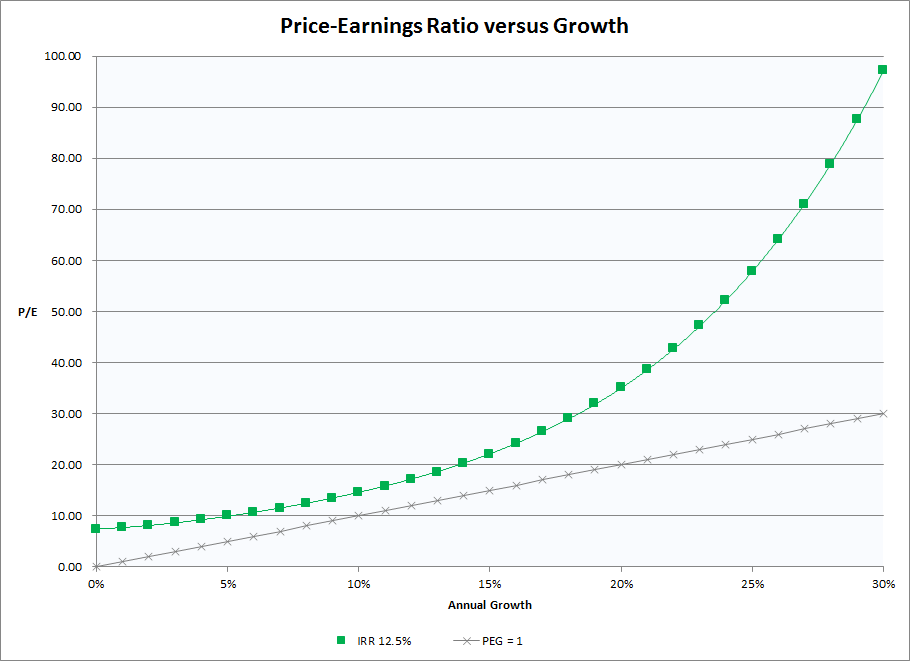# Price-Earnings versus Growth

A comment at lunch today about high price-earnings multiples got me started on one of my favorite topics: investment returns.

Most of us use the basic price-earnings ratio (PE or P/E) as a rough measure of how highly priced a stock is. The higher the P/E, the higher the risk.

But P/E only focuses on current earnings and ignores future growth which can make a huge difference to the return on your investment.

The PEG ratio, popularized by Peter Lynch in One Up On Wall Street, attempts to address this deficiency by dividing the price-earnings ratio by expected long-term growth rate of earnings.

• more than 1.0 is poor;
• less than 1.0 is good;
• less than 0.5 is excellent.

Comparing P/E to long-term growth is a step in the right direction but the PEG ratio has two notable deficiencies:

• It ignores dividends; and
• It assumes that the relationship between P/E and growth is linear.

### Dividends

It is fairly obvious that two stocks trading on the same P/E, and with the same expected long-term growth rate, do not present the same value if one pays regular dividends and the other does not. PEG can be adjusted to compensate for this deficiency, by adding the dividend yield to the expected growth rate. If we assume Computershare (ASX:CPU), for example, has a long-term growth rate of 10%, this should be adjusted to 12% to include the expected 2% dividend yield.

### P/E versus Growth

The relationship is not linear. Take a look at the graph below which compares P/E ratios on the vertical axis to Growth (including dividend yield) on the horizontal axis.The gray line plots stock prices at a PEG ratio of 1. A P/E of 10 intersects with growth at 10%, a P/E of 20 with growth at 20%, etc.

The green line plots an internal rate of return at 12.5% p.a. on investment. Here is a brief explanation of my calculation:

I project earnings of \$1 at varying growth rates for a period of 20 years. Then I discount this at 12.5% p.a. to arrive at a present value which equates to the P/E ratio (PV/\$1 earnings in Year 1). I assume an exit value of zero for two reasons: (a) to simplify the model; and (b) to compensate for declining growth rates over time. I can give you ten different alternatives but this seems the most effective treatment (and I am trying to avoid this reading like a PhD thesis).

The relationship between P/E and expected growth rate is clearly exponential. If we require an annual return on investment (ROI) of 12.5% :

• A growth rate of 15% would justify a P/E of 22; while
• A growth rate of 30% would justify a P/E of 97.

What is clear is that price may be dictated more by high expected earnings growth than by current earnings.

How do we estimate long-term earnings growth? With difficulty.

But this is the most important factor in determining a stock’s value so we need to make our best effort. Factors to consider include:

• Past revenue growth (earnings growth without corresponding revenue growth is difficult to sustain);
• Cash flow to fund future growth
• Market position
• Market growth or market saturation
• Market share
• Ability to withstand competition (rising profit margins are often a good pointer)

### Conclusion

The next time you look at a PE ratio, remember that earnings growth may be more important than current earnings.